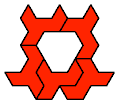# Polyiamond and Polyming Tiling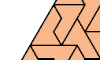Tiling a Triangle with a Polyiamond. Tile a triangular polyiamond with copies of a given polyiamond.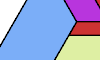Tiling a Triangle with a Scaled Polyiamond. Tile a triangular polyiamond with copies of a given polyiamond at various scales.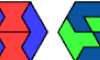Two-Hexiamond Balanced Hexagons. Tile a regular hexagon with two hexiamonds in equal quantities.Polyiamond Hexagon Tiling. Tile a straight or ragged hexagon with various polyiamonds.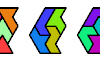Hexiamond Triplets. Arrange the 12 hexiamonds to form three congruent polyiamonds.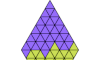Yin-Yang Diamonds. Arrange the 12 hexiamonds to cover a bi-colored diamond.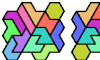Tiling a Polyhex with the 12 Hexiamonds. Arrange the 12 hexiamonds to form a polyhex.Similar Hexiamond Figures, 2–2–8. With the 12 hexiamonds, make three similar figures, one at double scale.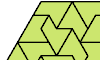Minimal Convex Polyiamond Tilings. With copies of a given polyiamond make the smallest convex polyiamond.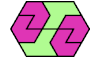Convex Polygons from Pairs of Polyiamonds. With copies of two given polyiamonds make the smallest convex polyiamond.Convex Polygons from Three Hexiamonds. With copies of three given hexiamonds make the smallest convex polyiamond.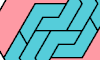Similar Polyiamonds Forming a Convex Shape. Arrange scaled copies of a polyiamond to make a convex polyiamond.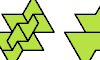Polyiamond Bireptiles. Join two copies of a polyiamond, then dissect the result into equal smaller copies of it.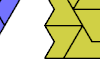Uniform Polyiamond Stacks. Arrange copies of a polyiamond to form a shape with equal, contiguous rows of cells with even length.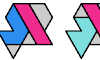Containing Pairs of Hexiamonds. Find the smallest polyiamonds that can contain every pair of distinct hexiamonds.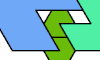Scaled Polyiamond Tetrads. Arrange four copies of a polyiamond at varying scales so that each borders the others.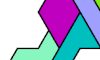Tiling a Shape with Ternary Symmetry with the Heptiamonds and the Tetrahexes. Tile a shape with 3-fold symmetry with all 24 heptiamonds, then with all 7 tetrahexes.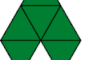The Lobster and the Snake. Four puzzles about the Lobster and Snake hexiamonds.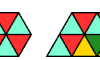Tiling a Convex Shape with a Polyming. Arrange copies of a polyming to form a minimally convex shape.

Back to Polyform Tiling < Polyform Curiosities
Col. George Sicherman [ HOME | MAIL ]